# Lecture 23: Stable Matchings

## Announcements

Reminders:

1. Final project submissions due Wednesday, 5/10 by 5:00pm
2. After final project submissions, peer review during finals week
• due Friday, 5/19 by 5:00pm

Class Next Monday (05/08)

• no lecture
• workshop/OH
• Oren and I will have last-minute help

## Overview

1. Stable Marriage Problem
2. Gale-Shapley Algorithm
3. Gale-Shapley Demo
4. Different Perspectives
• my research

## Internship Assignment Problem

In a small world…

• Four students: $a, b, c, d$
• Four internships: $A, B, C, D$

Question. How should we assign students to internships?

## Preferences

Agents have preferences in the form of a strict ranking of alternatives

• each student ranks available internships
• each internship ranks available students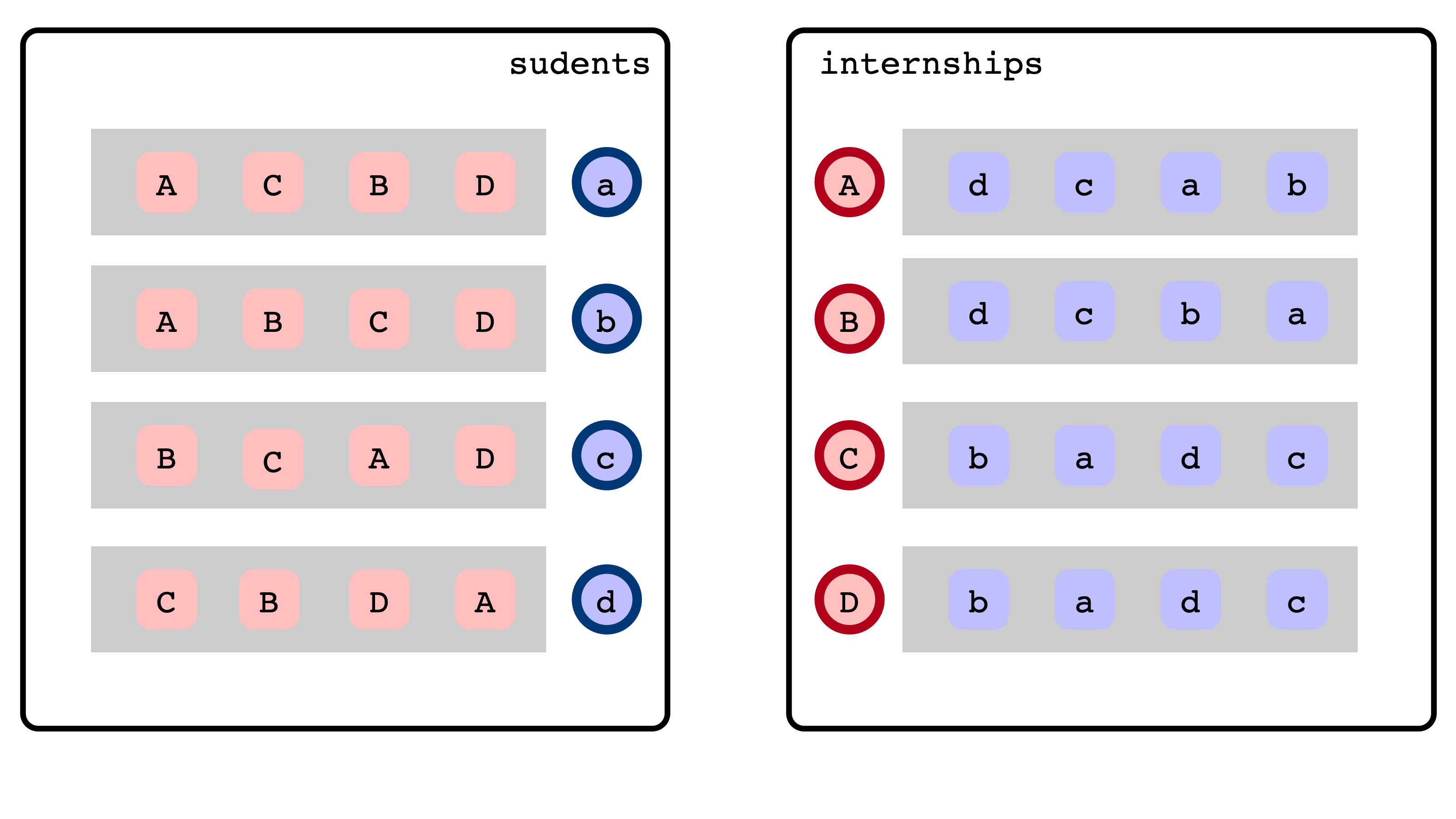## Question

How do we decide whether a matching “respects” agents’ preferences?## Blocking Pairs and Stability

Given:

• students, internships, preferences
• matching $M$

We say $(s, t)$ is a blocking pair if

1. $s$ and $t$ are not matched with each other
2. $s$ prefers $t$ to assigned internship in $M$
3. $t$ prefers $s$ to assigned student in $M$

## Blocking Pair Illustration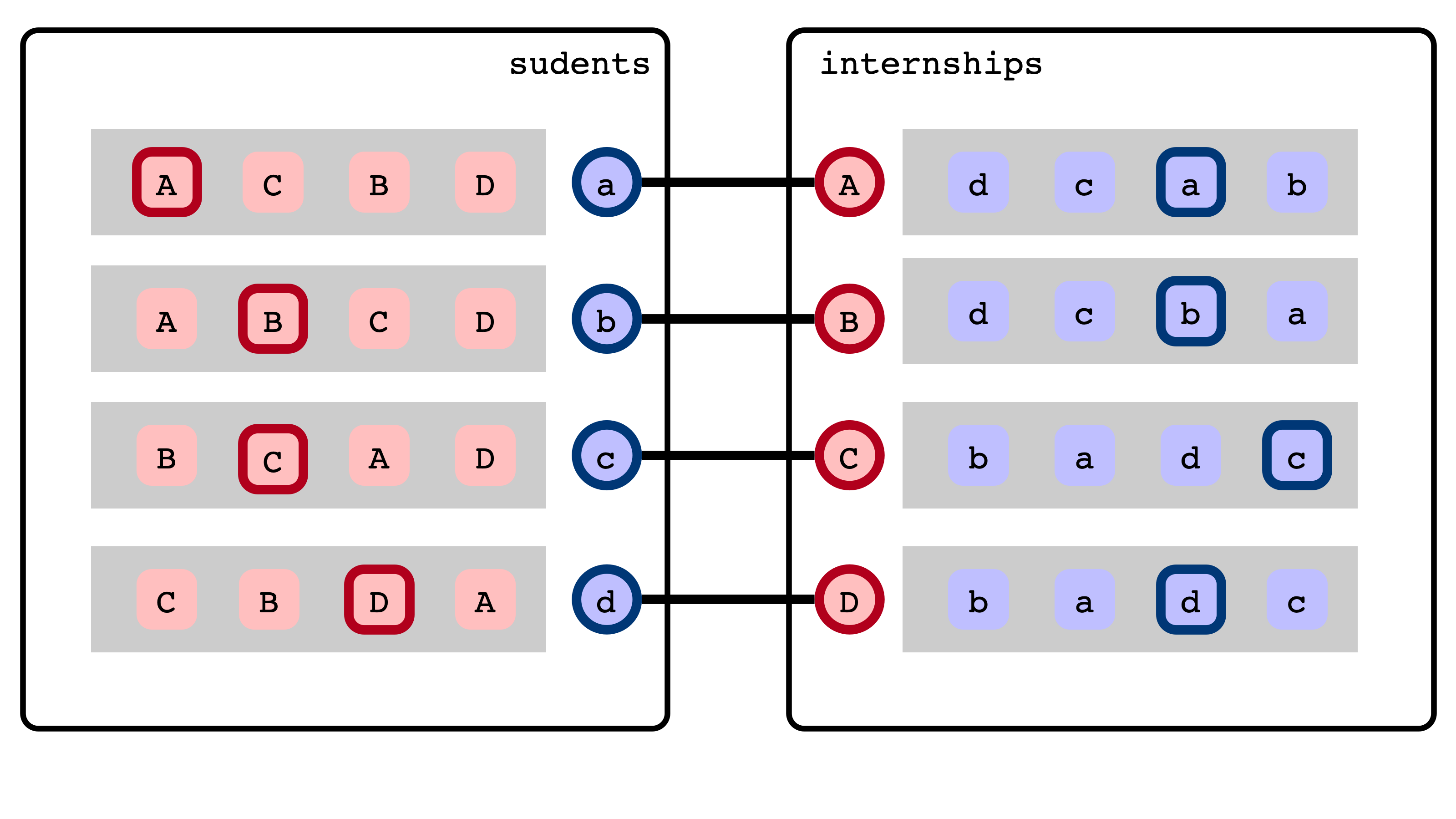## Blocking Pair Illustration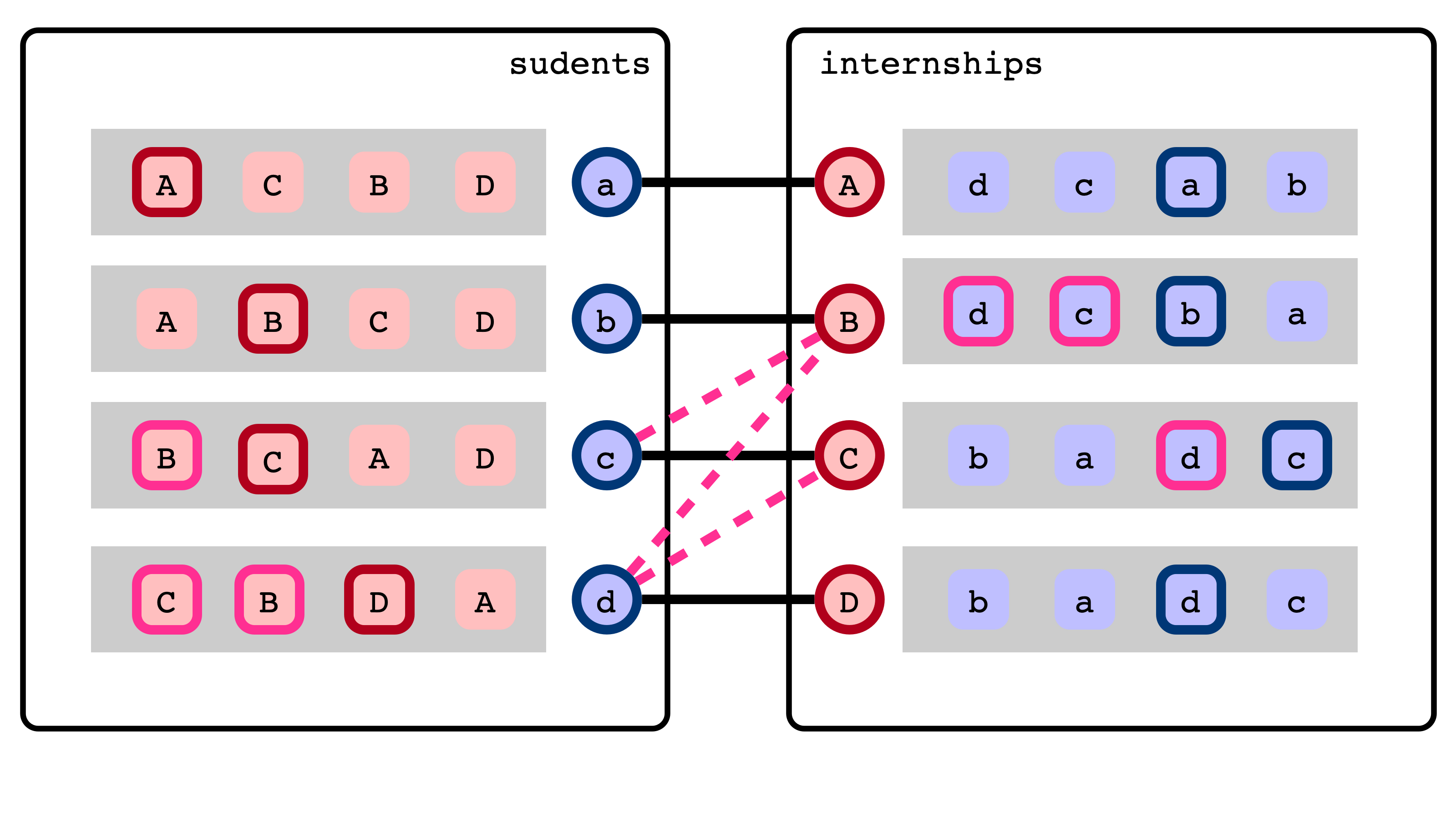## Stable Marriage Problem

Gale-Shapley 1962

Input:

• set of $n$ students
• set of $n$ interships
• for each student $s$, preference list ranking all internships
• for each internship $t$, preference list ranking all students

Output:

• a matching $M$ between students and internships
• $M$ is stable
• there are no blocking pairs

## Questions

1. Do stable matchings always exist?
• are there sets of preference lists for which there is no stable matching?
2. How can we find a stable matching (if one does exist)?

Theorem (Gale-Shapley 1962). Yes! Stable matchings always exist, and there is an efficient algorithm to find one.

## Gale-Shapley, Illustrated

• lec23-stable-matchings.zip

## Gale-Shapley Pseudocode

1. initially, all students/internships unmatched
2. while some student is unmatched
• for each unmatched student $s$,
• $s$ applies to next favorite internship
• for each internship $t$
• $t$ defers best applicant so far, rejects others
• rejected students unmatch

## Observations

1. Students apply sequentially in decreasing order of preference
• $s$ only applies to $t$ after $s$ has been rejected by all preferred internships
2. For each internship, deferred candidates are increasingly preferred
3. Once an internship receives an application, it stays matched

## Termination

Claim 1. Gale-Shapley terminates after at most $n (n - 1) + 1$ applications.

## Stability

Claim 2. When Gale-Shapley terminates, the resulting matching is stable.

## Conclusion

Theorem (Gale-Shapley, 1962). Every instance of the stable marriage problem admits a stable matching. If there are $n$ students and internships, a stable matching can be found in $O(n^2)$ time.

## Influence and Applications

• Introduced stability as key concept in economics
• 8,000+ papers spanning econ/cs/math
• 2012 Nobel Prize in economics (Roth and Shapley)
• stable allocations and mechanism design
• Applications:
1. matching med students with residencies

2. content delivery networks
3. kidney exchanges (variant)

## Influence on My Research

Stable matchings in a decentralized setting

1. Each agent is own computational entity
2. Agents must communicate in order to find a matching

## Question 1

Is it reasonable to assume all agents explicitly know their own preferences?

## Other Mechanisms

Do not assume preferences are explicitly known:

1. match-maker interacts with agents by performing queries
• a query is simply a yes/no (Boolean) function about preferences
• e.g., “Would you prefer to work for a large company, or a small company?”
2. match maker performs queries until enough information about preferences is elicited to determine a stable matching
• like “20 Questions”

Note. Gale-Shapley can be implemented with $O(n^2 \log n)$ queries.

## A Result

Theorem (Gonczarowski, Nisan, Ostrovsky, R–). Any mechanism that finds or verifies a stable matching uses $\Omega(n^2)$ queries in the worst case.

• finding/verifying stable matchings reveals a significant amount of information about preferences
• running time of Gale-Shapley is optimal, up to $\log n$ factor

## Further Implications

Finding “almost stable” matchings also requires $\Omega(n^2)$ queries.

• “early binding commitments” either lead to

1. instability
2. unraveling

# Implementation Notes

## Structure

1. Agent class for a student/internship
• has id, type, preference lists and current match
• compare proposals and reject
2. AgentVisualizer stores DOM elements to represent an Agent
3. SMInstance
• collections of students and internships
• generates random preferences, handles proposals/rejections
4. MatchingViewer keeps track of lines
5. GaleShapley steps through proposal/rejection rounds

## JavaScript Variable Assignment

What is wrong with this method?

this.receiveProposal = function (agentID) {
if (!this.prefList.includes(agentID)) return agentID;
if (this.curMatch == null) {
this.curMatch = agentID;
return null;
}
if (this.prefers(agentID, this.curMatch)) {
let rejected = this.curMatch;
curMatch = agentID;
return rejected;
}
return agentID;}


## Not Equivalent?

1. let a = 4
2. a = 4
3. var a = 4

## Dammit, JavaScript!

SomeClass = function () {
this.a = 4;

this.foo = function () {
a = 5;
...
a = 6;
}
}


## Drawing Preference Lists

The Setup. AgentVisualizer stores this.prefElts, an array of DOM elements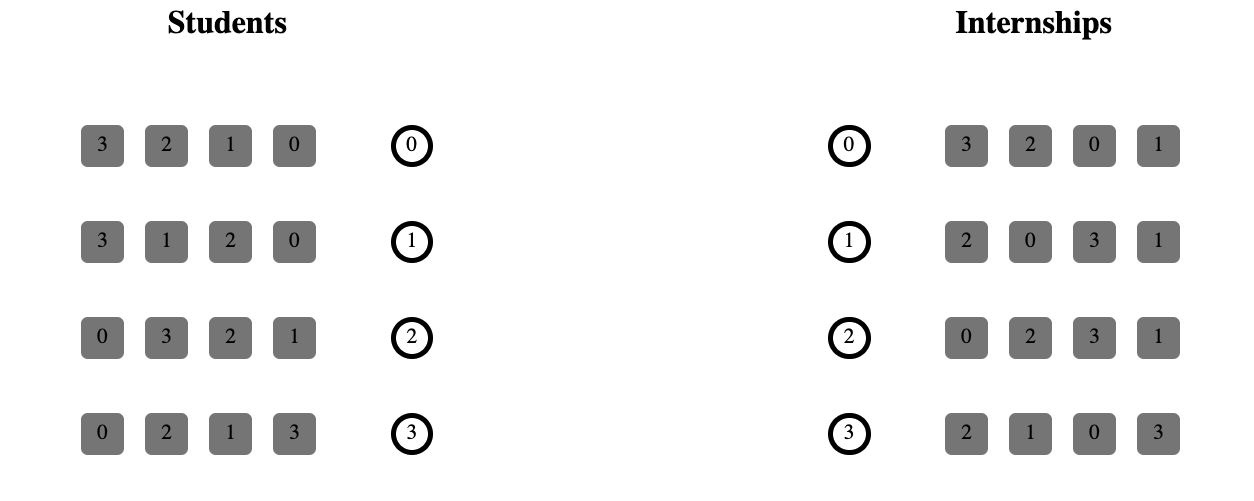## The Goal

Draw the elements in preference rank order

• should be easily modifiable (if prefs change)
• use a flexbox to handle the actual drawing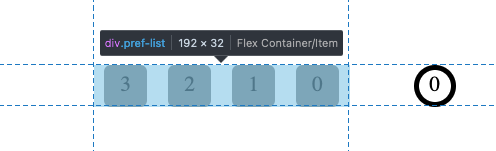## A Tool

You can associate data with DOM elements

• think a variable/value associated to each element

In JS, this can be done by setting the dataset attribute:

const pref = document.createElement("div");
pref.innerText = '' + id;
pref.dataset.rank = this.agent.prefList.indexOf(id);
pref.dataset.id = id;
this.prefs.appendChild(pref);
this.prefElts.push(pref);


## Sorting DOM Elements by Rank

this.sortPreferenceElements = function () {
for (let pe of this.prefElts) {
pe.dataset.rank = this.agent.prefList
.indexOf(Number(pe.dataset.id));
}

this.prefElts.sort((a, b) => a.dataset.rank - b.dataset.rank);

for (let elt of this.prefElts) {
this.prefs.appendChild(elt);
}}